# SUMIFS in Google Sheets to sum cells with multiple criteria (AND / OR logic)

The tutorial shows how to use the SUMIFS function in Google Spreadsheets to sum cells with multiple criteria. You will find a handful of real-life SUMIFS formula examples with AND as wells as OR criteria.

Google Spreadsheets provide two functions to conditionally sum cells, SUMIF and SUMIFS. Regular visitors of our blog are already familiar with SUMIF that we covered last week, and today we are going to have a closer look at its plural version.

The difference between SUMIF and SUMIFS is as follows:

SUMIF can add up cells based on just one condition. If it is what you want, please check out this tutorial: SUMIF in Google Sheets.

SUMIFS can sum cells based on two or more conditions. Further on in this tutorial, you fill find a simple explanation of SUMIFS syntax and formula examples for different data types.

## Google Sheets SUMIFS function - syntax & basic uses

The SUMIFS function is Google Sheets is designed to sum numbers with multiple conditions. Its arguments are as follows:

SUMIFS(sum_range, criteria_range1, criterion1, [criteria_range2, criterion2, …])

Where:

• Sum_range(required) - the range to be summed.
• Criteria_range1 (required) - the range of cells to be evaluated by criterion1.
• Criterion1 (required) - the condition to be met by cells in criteria_range1.
• Criteria_range2, criterion2, … (optional) - additional ranges and criteria to test.

### SUMIFS in Google Sheets - 3 things to remember

Using SUMIFS formulas in Google spreadsheets is quite easy. To prevent most common errors, just remember these three simple facts:

1. The SUMIFS function adds up only those cells that meet all conditions, i.e. all of the specified criteria are true for a cell. This is commonly referred to as AND logic.
2. Sum range and all criteria ranges should be equally sized, i.e. have the same number of rows and columns, otherwise your SUMIFS formula will throw a #VALUE error.
3. Depending on the nature of your conditions, build your criteria appropriately:
• Text, wildcard characters and dates should be enclosed in quotation marks like "apples" or "*" or "3/15/2018".
• Numbers and cell references are not enclosed in quotation marks when used on their own.
• Logical operators with numbers, dates or text must be enclosed in double quotes like ">200", ">3/11/2018" or "<>apples" .
• Logical operators used together with cell references and other functions require the quotes to begin a text string and ampersand to concatenate and finish the string off. For example: ">"&B1 or ">="&TODAY().

Okay, that's enough theory, let's build some useful formulas already!

## How to sum with multiple criteria (AND logic)

When it comes to summing cells with multiple criteria, you may find a lot of different suggestions in Google groups and other user communities.

Let's say, you have a list of items in column A, amounts in column B, and delivery status in column C. Your goal is to get a total for a specific item with a certain delivery status, for example "apples" that are "delivered". How would you approach the task?

You may be surprised to know that many people still use array formulas for this purpose. For example, this one:

`=ARRAYFORMULA(SUMPRODUCT((A6:A14="apples")*(C6:C14="delivered")*(B6:B14)))`

What's wrong with this formula, you may ask me. Nothing except that it's excessively complex, difficult to understand and debug. It's like using a sledgehammer to crack a nut. Google Sheets SUMIFS function provides an easier way!

So, let's go ahead and define our arguments:

• Column A should contain "apples". Assuming we have data in rows 6 to 14, our criteria_range1 is A6:A14, and criterion1 is "apples".
• Column C should contain "delivered". Meaning, our criteria_range2 is C6:C14, and criterion2 is "delivered".
• Numbers to sum are in column B, so our sum_range is B6:B14.

Putting the arguments together, we get this simple formula:

`=SUMIFS(B6:B14, A6:A14, "apples", C6:C14,"delivered")`

To add more flexibility, you can input both criteria in separate cells, say B1 and B2, and refer to those cells:

`=SUMIFS(B6:B14, A6:A14, B1, C6:C14, B2)`

As shown in the screenshot below, our SUMIFS formula works perfectly!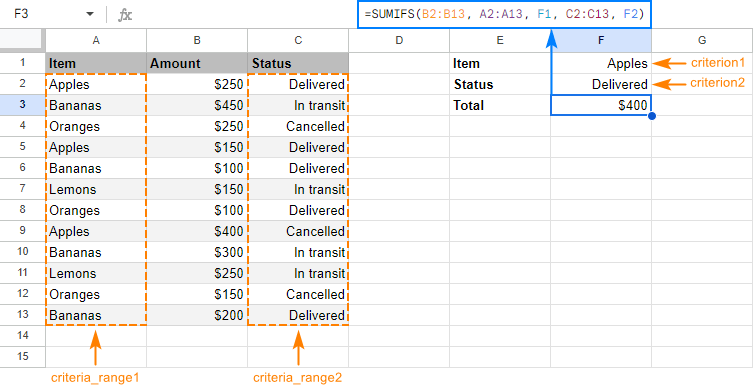Taking the example further, let's see how to use SUMIFS with other criteria types.

### SUMIFS with logical operators

When your conditions are numbers or dates, use the comparison operators to express the criteria:

• equal to (= or omitted)
• not equal to (<>)
• greater than (>)
• less than (<)
• greater than or equal to (>=)
• less than or equal to (<=)

For example, to add up amounts in B6:B14 that are greater than or equal to 200 and delivered before 1-Apr-2018, use this formula:

`=SUMIFS(B6:B14,B6:B14,">=200", C6:C14, "<4/1/2018")`

In case you want to replace actual values with references to cells holding your criteria, please remember to enclose the logical operator in quotation marks and to concatenate the cell reference by using an ampersand:

`=SUMIFS(B6:B14,B6:B14,">="&B1, C6:C14, "<"&B2)`

The screenshot below shows the result: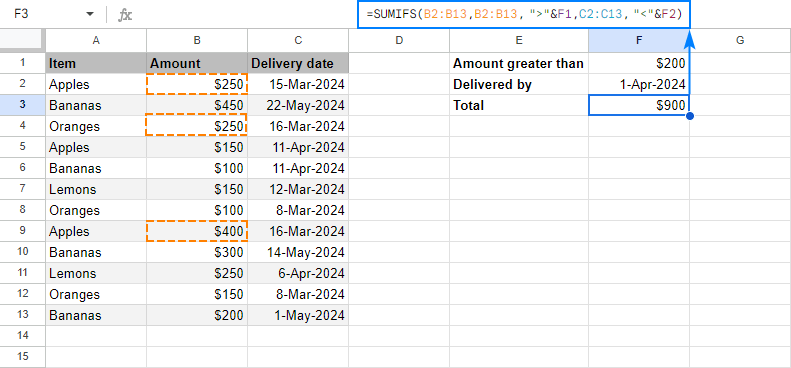### SUMIFS with other functions

In some situations, the conditions may depend on the results returned by some other functions. In this case, embed those functions in the criterion arguments of your SUMIFS formula.

As an example, let's sum "apples" that are "delivered". In other words, the delivery date is up to and including today, so we concatenate the "less than or equal to" operator (<=) with the TODAY() function:

`=SUMIFS(B6:B14,A6:A14, B2,C6:C14, "<="&TODAY())`

And get the following result: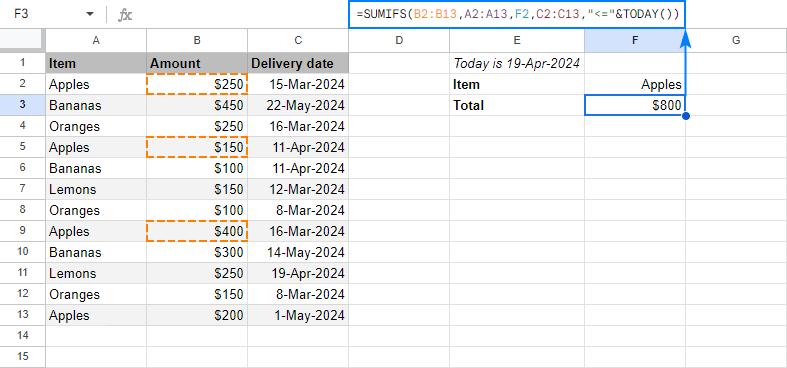### SUMIFS with blank and non-blank cells

To sum numbers in one column depending on whether a cell in another column is empty or not empty, use one of the following criteria:

• "=" to sum cells that are completely blank, i.e. contain absolutely nothing.
• "" to sum empty cells including zero length strings.
• "<>" to sum non-blank cells including zero length strings.

In case the Delivery date column contains some gaps, you may want to sum only those "apples" for which the delivery date is set, i.e. a cell in column C is not empty. The task can be accomplished with this formula:

`=SUMIFS(B6:B14,A6:A14,"apples" ,C6:C14, "<>")`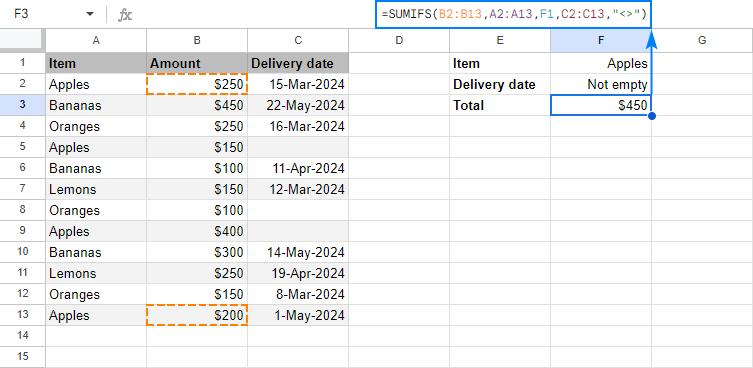## How to sum with multiple criteria (OR logic)

As you already know, by default, Google Sheets SUMIFS function works with AND logic - all conditions must match to be summed. In some situations, however, you may need to conditionally sum cells with OR logic, when any of the specified criteria is true. Below you will find a few possible ways to do this.

### SUMIF with OR criterion (multiple criteria in the same column)

To sum cells with only a couple of criteria in the same column, you can simply add two SUMIF functions together.

For example, to sum the amounts in column B if column A is either "apples" or "bananas", use the SUMIF() + SUMIF() combination in its simplest form:

`=SUMIF(A:A,"apples",B:B) + SUMIF(A:A,"bananas",B:B)`

If you have three or more criteria, you may be looking for a more compact formula. For this, include the items in an array constant (aka inline array), use ArrayFormula to get a subtotal for each item, and wrap the whole construction into SUM() to add the subtotals together. For eample:

`=SUM(ARRAYFORMULA(SUMIF(A6:A14, {"apples", "bananas", "lemons"}, B6:B14)))`

Instead of "hard-coding" the items in an array constant, you can enter them in individual cells, and include cell references in the array (in case of non-contiguous cells) or supply a range (in case of contiguous cells).

To better show you that our SUMIF formula works in exact accordance with the specified OR criteria, I've narrowed down the list to two items:

`=SUM(ARRAYFORMULA(SUMIF(A6:A14, {B1, B2}, B6:B14)))`

Or

`=SUM(ARRAYFORMULA(SUMIF(A6:A14, B1:B2, B6:B14)))`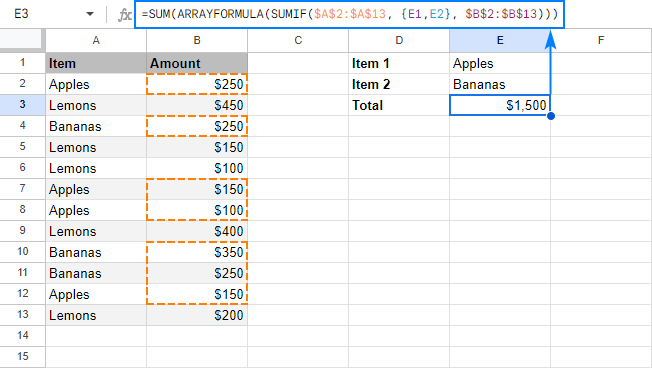Instead of ArrayFormula, you can add up subtotals with the SUMPRODUCT function. For a short list of items, you can put it this way:

`=SUMPRODUCT((A6:A14="apples") + (A6:A14="bananas"), B6:B14)`

For multiple OR criteria (three or more items), you'd better use this syntax:

`=SUMPRODUCT((A8:A16={"apples", "bananas"}) * B8:B16)`

Replace the array elements with cells references, and you will get the most compact formula to sum cells with multiple OR criteria ever!

`=SUMPRODUCT((A8:A16={B1, B2}) * B8:B16)`

The screenshot below shows the result: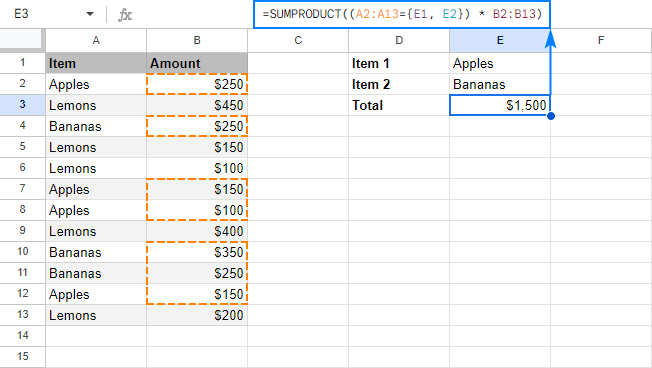Four different formulas, the same result. Which one to use is the matter of your personal preference :)

### SUMIF with OR criterion and results in different cells

If you'd like to have a subtotal for each item in a separate cell, take an array SUMIF formula discussed above, adjust the references, and cut off the SUM() part:

`=ARRAYFORMULA(SUMIF(A5:A13, {"apples", "bananas", "lemons"}, B5:B13))`

This will give you a sum for each item in a separate cell as shown in the screenshot below: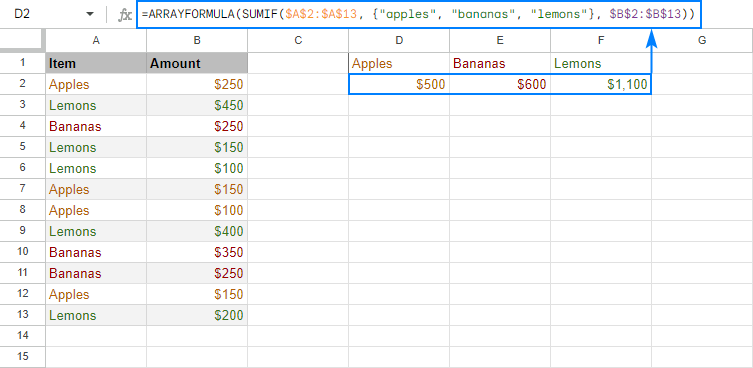For the sake of clarity, you enter the formula only in the leftmost cell (A2 in this example), and Google Sheets will put the results into as many cells as many items there are in your array constant. Just make sure you have enough empty cells to the right, otherwise some of your data may be overwritten.

If you'd rather have subtotals in a column, then separate the array elements with semicolons to make a vertical array:

`=ARRAYFORMULA(SUMIF(A2:A10, {"apples"; "bananas"; "lemons"}, B2:B10))`

And the results will be output vertically in a column: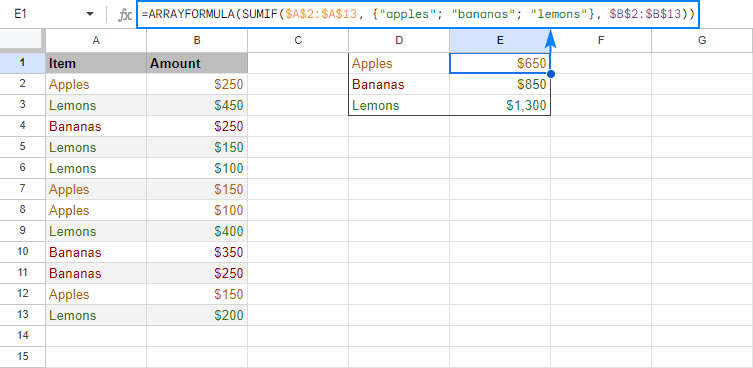Tip. As usual, you can make your formula more flexible by replacing a hard-coded array with a range reference. That way, your users could type any items in the predefined cells, and you won't have to worry about updating your formula. For example, the formula shown in the screenshot above, can take the following shape:
`=ARRAYFORMULA(SUMIF(A2:A10, D1:D3, B2:B10))`

### SUMIFS with OR criteria (multiple criteria in different columns)

This example shows how to sum numbers with several sets of conditions determined by the following logic.

• Within each set, all conditions must be true (AND logic)
• A cell is summed if any set of conditions is true (OR logic)

To make things easier to understand, please consider the following example. In our sample data set, supposing you want to sum amounts in column B if column A contains either "apples" OR "oranges" AND the delivery date in column C is "16-Mar-2018".

The most obvious way is to make two SUMIFS formulas to sum "apples" and "oranges" separately, and then add up the results:

```=SUMIFS(B6:B14, A6:A14, "apples", C6:C14, "16-Mar-2018") + SUMIFS(B6:B14, A6:A14, "oranges", C6:C14, "16-Mar-2018")```

Or, you can enter your criteria in some cells, as shown in the screenshot below: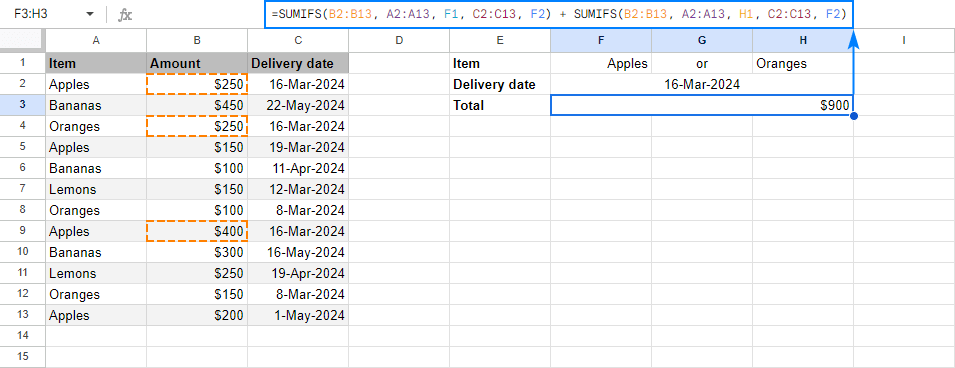Regrettably, Google Sheets do not allow expressing multiple OR conditions using array constants, therefore we cannot make a plural version of our SUMIF with OR criteria formula. Luckily, there is another way to achieve the same result with a bit shorter formula - the SUPRODUCT function.

As an example, let's enter the desired delivery date (AND criterion) in B2 and items (OR criteria) in contiguous cells, say B1 and C1. Now, you can use the following formula to sum numbers in B6:B14 based on the above criteria:

`=(SUMPRODUCT(--(C6:C14=B2), (--(ISNUMBER(MATCH(A6:A14, B1:C1, 0)))), B6:B14))`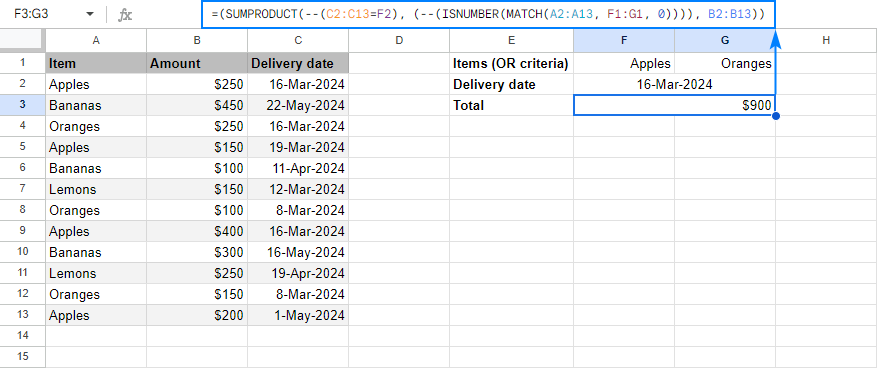#### How this formula works

Working from the inside out, here's what you do:

• Check if an item is "apple" or "orange":
`ISNUMBER(MATCH(A6:A14, B1:C1,0))`

As the result, you'll get an array or TRUE and FALSE values (TRUE if any of the specified criteria is met, FALSE if none of the criteria is met).

• Compare a list of dates with the target date: C6:C14=B2

Instead of a cell reference, you can enter the date directly in a formula by using DATEVALUE or DATE function. For example, C6:C14=DATEVALUE("3/16/2018") or C6:C14=DATE(2018,3,16)

In this formula, however, you cannot compare a range with a date directly, like C6:C14="3/16/2018" or C6:C14="16-Mar-2018", because you'd be comparing a cell with a date to a text string. Google Sheets won't understand that and you will most likely get a zero result.

If done correctly, this comparison will give you another array or TRUE and FALSE.

• Use the double unary operator (--) to coerce both arrays of TRUE and FALSE to 1 and 0, respectively.
• Supply the range to sum: B6:B14

SUMPRODUCT will first multiply the elements of 3 arrays (two arrays of 0's and 1's and an array of numbers in B6:B14), and then sum the products. Because multiplying by 0 always yields 0, only the cells that have 1 in the first two arrays will "survive". As the result, only the amounts with the specified delivery date and item names will be summed.

This is how you use SUMIFS in Google Sheets to sum cells with multiple conditions. To have a closer look at the formulas discussed in this tutorial, I invite you to open our SUMIFS Google Sheets Examples. I thank you for reading and hope to see you on our blog next week!

## You may also be interested in

1. Hi, is it possible to include importrange with sumif? I wanted to sum data from a spreadsheet, criteria is date and sum range is amount. I would like this to be entered into a different spreadsheet.

• Hi Peesot,

Well, at the moment SUMIF does work with IMPORTRANGE but only if first you transfer the required range with a separate IMPORTRANGE and give the function permissions to access another spreadsheet. Otherwise, your SUMIF will return 0.

You will find the tutorial on IMPORTRANGE in this blog post.

2. Hi,
I'm hoping you can help me with a formula.
arrayformula with sum function a1+a2,a3+a4,a5+a6..................pair 2-2-2
and a1+a2+a3,a4+a5+a6,a7+a8+a9....................Pair 3-3-3

• Hi Ravi,

3. I'm hoping you can help! I've read through all of the queries above hoping someone would be trying to do the same thing as me and have searched other forums as well.

Basically, I want a sum of the values in column J the value in column D is "Law" and the value in column F is "2021". The formula below returns a result of 0. What am I doing wrong?

=SUMIF(AND('2021 EO Sales'!D:D,"Law",'2021 EO Sales'!F:F,"2021"),'2021 EO Sales'!J:J)

• Hello Katy,

You're trying to sum based on two conditions using SUMIF. The SUMIF function adds up based on one condition only. The AND function won't help there.
To sum by several conditions, you should use SUMIFS instead. It's the function described in this blog post (see above). Please look through it closely and you will be able to build the correct formula.

4. Hi,
I'm hoping you can help me with a formula. I have attempted to look through many forums but not quite been able to find what I'm looking for.
I am attempting to sum multiple columns with multiple criteria that also relies on criteria from other columns and one of those has multiple criteria as well.
Below is the outcome I am looking for
Desired result for cell I18
SUM all columns S,W,AA,AE,AI,AM
IF columns
H = AA
K = W
J = Jul19|Aug19|Sep19|Oct19|Nov19|Dec19|Jan20|Feb20|Mar20|Apr20|May20|Jun20
Please see below the link to the spreadsheet - this could be restricted so please let me know if you are unable to open
I hope to hear from you. I am desperate!

• Hi Nicole,

Note. We keep that Google account for file sharing only, please do not email there. Once you share the file, just confirm by replying here.
Thank you.

5. Sumifs is very heard

6. we have 3 columns as a "sum_range" .. but the results only shows 2 columns being count..

any solution?

thank you

One SUMIFS formula takes only one sum_range at a time. For us to be able to advise you anything, please share the exact formula you're using.

• where should we send the link to ?!
it seems prohibited to post it here..

I can see that you refer to 3 columns with one criteria range. Please specify if you're trying to SUM the total when the name from S5 appears in at least one column (E-G) or in all three columns.

If you need something other than that, please add the sheet with an example of what you're trying to get.

• Thankyou so very2 much for responding, i'm sorry for the late reply..

The idea is to get the total work for 1 person on certain date..
They are working as team (of 2 or 3) .. depends on the jobs load..
.. only 2 out of 3 columns get counted / recognized

i'll share the latest Sheet soon..
Thank you for your assistant .. i'm so happy for this.. :)

Thank you for editing the spreadsheet.

I added correct formulas to the column V and highlighted them green, please take a look. I had to add up multiple SUM functions since SUMIF doesn't work the way you need. SUMIFS also won't help, since it will look for names in all 3 columns at once.

i will look into it a s a p..

7. Hi,

I'm trying to use the SUMIF/SUMIFS OR criterion with wildcards i.e. as in your example above but

=SUM(ARRAYFORMULA(SUMIF(A6:A14, {"*apples*", "*bananas*", "*lemons*"}, B6:B14)))

I can only seem to get this to work with a SUMIF and not a SUMIFS - when I translate the above formula into its SUMIFS equivalent:

=SUM(ARRAYFORMULA(SUMIFS(B6:B14, A6:A14, {"*apples*", "*bananas*", "*lemons*"})))

the formula does not work (it only respects the first criterion "*apples*".

Am I doing something wrong? Or is there a reason this wouldn't work with SUMIFS?

Thanks!

• Hi Michael,

As Svetlana stated above, Google Sheets SUMIFS function works with AND logic only. That is the reason why SUMIF is used for the OR logic instead.
So I'm afraid you won't make SUMIFS work and will have to go with either SUMIF or SUMPRODUCT.

8. =SUMIFS('p_s MastNum'!\$F:\$HF,'p_s MastNum'!\$B:\$B,\$D\$1,'p_s MastNum'!\$C:\$C,\$F\$1,'p_s MastNum'!\$E:\$E,\$B\$19,'p_s MastNum'!\$F\$3:\$HF\$3,\$A20)

is my formula

I get a #Value error.

If I remove the last criteria and use \$F:\$F as the range, it works. However, I want it to return/add the values in row 1 & 2. It is another filter I need to use.

In Sheet "p_s MastNum"
Row 1 & 2 are names
Row 3 is a helper row joining the two names with a " "
Col B = Month each row has a value
Col C = Year each row has a value
Col E = 1 of 7 headers in each row. There are more than 1 header in the column

• Hello Rain,

For me to be able to help you, please share an editable copy of your spreadsheet with us (support@apps4gs.com) with 2 sheets: 1) source data with a formula, 2) the result you expect to get.
Note. We keep that Google account for file sharing only, please do not email there. Once you share the file, just confirm by replying here.

9. Gracias

10. Im trying to do a formula, not sure if the ones above are suitable - essentially I want to have a total that is increased/deducted by whether or not a number in a cell has a yes or no in the cell next to it.

Basically I have a "bills paid out or to be paid out" section listed and then want to select yes or no next to it to say if its been paid yet or not which will then auto update the "whats left to pay out this month". so cell A1 - Car Insurance B1 - £153 C1 Yes - by changing it to yes, it doesnt calculate this in total for the month, it excludes it. but if it said no, it will include it in the total left to go out?

Hope this makes sense!

• Hello!
If I understand your task correctly, the following formula should work for you:

=SUMIFS(B1:B10,C1:C10,"No")

I hope this will help

11. Hi Natalia,
That worked perfectly!
Thank you so much for the help. Much appreciated.

12. Hi,
I have check boxes in column D rows 32-160, Numbers in column E rows 32-160, and a drop down box with numbers in column I rows 32-160.
In G19 I'm trying to enter a formula that will give me the number in each row of E multiplied by the number in corresponding row of I, if the check box in column D is checked. Then divide the resulting number by 16.
Below is the formula that I have been playing with.
=sumif(D32:D160, true, (E32*I32)/16:(E160*I160)/16)

• Hi Roger,

I'm afraid your formula won't work since SUMIF requires its third argument to be a range. As for your task, I believe it's better to use the IF function instead:
=ArrayFormula(IF(\$D\$32:\$D\$160=TRUE,\$E\$32:\$E\$160*\$I\$32:\$I\$160,""))

• Hi Natalia,
I tried your formula but am still having issues.
I see what your formula does. I think I missed one thing. I want the total of my results to be in G19.

I get the following error message:
Array result was not expanded because it would overwrite data in G20.

• Hi Roger,

The thing is that ArrayFormula processes the entire column and returns the result for each row at once. The contents of G20 (and below) doesn't allow the formula to return the outcome. Once you clear those cells, you will see the results. Try pasting the formula to an empty column – you'll see what the formula returns exactly.

If you need the total of those, just wrap the formula in SUM:
=SUM(ArrayFormula(IF(\$D\$32:\$D\$160=TRUE,\$E\$32:\$E\$160*\$I\$32:\$I\$160,"")))

13. i would like to add like this
A B
2 8
5
7
6
8
10
14
15
17
12
14
i want to add top values with respect to cell reference B.if it is between 5-9 than add top 5 values in A, if B is between 11-15 than add top 10 values in A. Please help me.

• Hello Rajveersingh,

Is it only one top cell in column B that you need to check? Or will other cells in column B have numbers to check as well?

14. Thank you! you're awesome!

15. Hi there
I am struggling with a formula, and hope you can help me to corect it.
SUMIFS(K2:K46;F2:F46;Q2; OM(SUM(N2:N46)>0))
I am need the formula to add all values in column K, for the names in column F which equals to the cell Q2 ----
But only if at the same time all the related values in row N are >0 (with related values do I mean the rows with the same name as in Q2)....
tried it with many alternatives, but did not succed, therefore did I try to summ up the values... And now do totaly give up and beg for your support

Thanks a lot
Fatos

• I am happy with the first part of the equation (to summ/add up values in cloumn K if the text in column F = text in cell Q2
but I really do not know how to combine, or even have a seperate equation for the second part, also same condition for text column F (=text in cell Q2) applies here for the range of rows in column N, but only if the value in those do not contain 0 aka are >0.

Looking forward to hear a solution
Thank you

• Hi Fatos,
If I understand your task correctly, this formula should do the trick:
=SUMIFS(K2:K46,F2:F46,Q2,N2:N46,">0")

Please look through this part of the article to understand how this formula works.

• Hi Natalia,

Thank you! In the past couple of days I came also to the same formula, but unfortunately it gives me not the result I need. So my fault, I formulated wrongly. I was not very clear I am afraid. I want the formula to calculate the total, or give me a 'yes' if both equations are ok
also F2:F46=Q2
AND All (the related) rows in column N are >0
So a 'yes' if both equations are met, a 'no' if there is even a single "0" in the rows of column N (but only for the rows there first part of equation (F2:F46;Q2)

So sorry, I am trying now for over a week, and can simply not find the solution.
Like written before, it is even ok if I need the 'split' the formula in 2-3 parts and do first one part in a cell, then another etc.... to reach the result

Have a great day!

• Hi Fatos,

Would you mind sharing a small sample spreadsheet with us (support@apps4gs.com) with 2 sheets: a sample of your data and the result you expect to get? I kindly ask you to shorten the tables to 10-20 rows. If you have confidential information there, you can replace it with some irrelevant data, just keep the format.

Note. We keep that Google account for file sharing only and don't monitor its Inbox. Please do not email there. Once you share the file, just confirm by replying here. I'll look into it and do my best to help.

• Hi Natalia,

Thank you so much. I've shared the file "Exempel Fatos" with you
Thanks again & kind regards

• Hi Fatos,

Thank you, I've got the file. I'll look into it as soon as possible.

• Hi Fatos,

I've looked into your file. Here's a formula you can use next to your additional column with unique names:
=IF(MINIFS(\$N\$2:\$N\$18;\$F\$2:\$F\$18;\$Q2)=0;"No";"Yes")

16. Month Job Hrs
20-Mar 40 10
20-Mar 60 12
20-Mar 10 5
20-Mar 15
20-Apr 50 12
21-Apr 45 8
22-Apr 10

20-Mar 20-Apr
No. of Job
No. of Hr I need a formula that will sum values from column C with the following criteria
1. sum all hours for particular month with values in column B

• Hello Dante,

Assuming the table covers 10 rows, here's the formula to sum all numbers for March:
=ArrayFormula(SUMIFS(C2:C10,MONTH(\$A\$2:\$A\$10),3,B2:B10,"<>"&""))

You just need to adjust the ranges (to your number of rows) and change that number 3 that stands for March to a number of your month of interest.

17. Hello! I love your content and I'm almost there with my formula :X but almost means still not.
How to use those IF to create a formula like this.

I have A, B, C.. columns
I would like to make specific operation 'plus' or 'minus' bases on a cell indication.

ANy idea?

• Hello Florian,

if I get it correctly and you need not only to sum but also subtract, try using the IF function instead.

18. Awsomely explained (and in a "human" language!!) Excellent examples and super useful! Thanks!

19. Help.

It's just not working with the SUMIFS
I've got this:
=sumifs(uren!\$E\$4:\$E ; uren!\$A\$4:\$A ; C2 ; uren!\$D\$4:\$D ; \$A3 )

uren!\$E\$4:\$E = referring to other tab, the sum up the hours
uren!\$A\$4:\$A ; C2 = saying search for c2 this range on the other tab
uren!\$D\$4:\$D ; \$A3 = saying search for c2 this range on the other tab

What do I not see???? I checked for so many times. And the cells seem to be correct.
It doesn't give an error, but keeps giving me a 0

• Hello Wouter,

You wrote: "uren!\$D\$4:\$D ; \$A3 = saying search for c2 this range on the other tab"
Actually, it searches for A3 in this range. Could this be the problem? Change A3 to C2 or adjust whatever lies in A3.

20. Hi Natalia and thank you for these helpful tutorials.
I would like to compare two text columns (on seperate sheets) lets say column A:A on sheet1 with column J:J on sheet 2. I also have a column B:B on sheet 1 with numbers. So when there is a match between Sheet1!A:A and Sheet2!J:J i would like to calculate the sum of the B:B correspondant on sheet1 . I hope it's clear ^^
Can you help me please ?

• Hi David,

This tutorial is actually Svetlana's, but I still thank you for your feedback :)

We have an article describing ways to compare data in different tables in Google Sheets, please have a look. You may find the solution to include to your SUMIFS there.

21. I'm having trouble finding a way to sum the exact same cells across 100+ worksheets. In this case, I'd have the worksheets all named something similar for the first part of the string (i.e "Submissions_From_Field_"Initials) while Initials will vary for each submitter. Is there a way to concatenate the worksheet name so that all of these can be included when I sum B3 across all worksheets and then won't have to type and open 100+ worksheets to get sum across all?

22. Hello,

I received a formula from someone else, but I need to modify it by 1 cell.

Original formula (works perfectly, but I'm unable to modify it myself):
=SUM(ArrayFormula(if(mod(sequence(1,COLUMNS(E6:6)),6)=1,E6:6)))

This formula was set up to SUM every 6th column, row 6 beginning from E6, but I need to modify it to begin at F6 instead. Simply changing the formula from "E6:6" to "F6:6" returns the "#ERROR!" sign and I can't figure this out.

I'd like to SUM F6, L6, R6, X6, AD6, etc. (Every 6th column). Plus I'll be routinely adding and deleting columns by groups of 6.

Columns A-C are permanent and are never deleted or added to.
Ex. Deleting columns D-I, which would delete F6

This formula worked perfectly for beginning at E6, but I cannot seem to simply change it to F6 as I'm met with "#ERROR!". What am I not understanding that makes it so "E6:6" cannot simply be replaced with "F6:6"?

Any help is much appreciated and I can't thank you enough!

• Hello,

The formula works on my side if I simply replace E with F.
You can try another formula instead:
=SUMPRODUCT((MOD(COLUMN(\$A6:6)-COLUMN(\$A6)+1,6)=0)*(\$A6:6))

23. Hi
=SUM(E9:E13)-'Cash Paid Out'!E27 how do I add a % calc to the first bit? e.g. E24/100*19

Many thanks

Mike

• Hi Mike,

I'm sorry I'm not getting your exact task. Could you please specify what you're trying to count? A percentage of the total? Percentage increase? If so, you may find this blog post useful.
Otherwise, please describe the task in more detail and I'll try to help.

24. Thank you ... this article was a life-saver and really helped bring my GSheet to life.
Appreciate the effort to educate others.

25. Looking for help with this:
IF SUM(H60,H75)>86.66, 43.33-SUM(H60,H75), 0)
• where H60 to H75 are a row of decimal values that shouldn't exceed 86.66
• if they exceed 43.33 the amount should be reduced by the difference (86.66-SUM(H60,H75))
For some reason this formula doesn't work. Is it syntax?

• JR,

=IF(SUM(H60:H75)>86.66,43.33-SUM(H60:H75),0)

26. And Thank You

• Hello Matt,

we have a tutorial on the basics of drop-downs in Google Sheets here. You may gain some ideas for your task there.

We could also try and help you out, but for that, I have to ask you to share your sample spreadsheet with us (support@4-bits.com) with your example data and the result you expect to get. Once you share the file, just confirm by replying here. Thank you.

27. I have a Google sheets workbook that utilizes a cover sheet which picks up data from subsequent sheets within the book. On the cover sheet I want to add a drop-down menu that will change the data values depending on the item listen from the drop-down list.

For example I want to see sales revenue data by country. Let's call it US, China, and Singapore. Within each sheet is a formula pulling in data for a hardcoded country, such as "US". I want to make this dynamic and changed based on the drop-down menu selection made. So if China is picked the SUMIFS will start looking for China.

Thoughts?

28. Dear ablebits.com Team,
How to use sumifs () function between multiple Google sheet files ?!
I want a file just to display the results from the data of other files!
For example: File A will use SUMIFS functions to add data from files B, C, D ...

• Dear Taieuro,

referencing another spreadsheet is not that easy in Google Sheets. You should use the IMPORTRANGE function to do that.

But since SUMIF requires pure ranges, it won't work with IMPORTRANGE. You will have to use SUMPRODUCT instead.

29. Thanks for this great explanation!
I think this is close to what I need; however, I'm having trouble with a nested quantity.
I have 3 sheets: Responses (Static Data), List (Static Data), Compiled (Static with Dynamic Values/formulas)
All columns are ranges with the respective names.
Responses (Static) contains the following "columns/ranges": RespDate, RespItem, RespQty
All Responses data could be duplicate entries.
List (Static) contains: ListItem, ListWeight
All ListItem entries are unique
Compiled (Static with Dynamic) contains: CompDate (Static), CompWeightTotal (Dynamic)
All CompDate entries are unique
I want to display the total weight of all items (CompWeightTotal) for each specific date (CompDate) in the Compiled Sheet.
Step 1: Get the Sum of RespQty of each specific item for that specific date.
Step 2: Multiply the sum of the Qty for that item for that specific date by the ListWeight.
Step 3: Get the Sum of all weights for that specific date.

I have part of Step 1; however, it is a general sum of RespQty: SUMIF(RespDate,A2,RespQty) where "A2" is a date. If 2 entries exist for this date and each entry had RespQty of 2, the returned value is 4.
I need to figure out how to count the quantities of a specific item and then multiply this sum to the weight of the specific item found in "List".

Maybe I should be using an array formula?

30. Has google changed the way SUMIF works? Sheets now says the order of operators is (Range,Criteria,CriteriaRange) which is different from what's described here, and Sheets also says that it can only take three operators, so it won't accept a Criteria2 and CriteriaRange2.

31. Hi All!
How can I do a NOT statement...
=SUMIFS( Range, Criteria Range, NOT "A")

Robert

• Hi Robert,

32. Can I use this to count checkboxes?
If so, what is the function?

• To count checkboxes, you need to use the COUNTIF function. You can learn more about it here.

Since Google sees checkboxes as TRUE (checked) or FALSE (unchecked), your formula should be like this:
=COUNTIF(A2:A10,TRUE)
where A2:A10 is the range with checkboxes and you count those that are checked. To count those unchecked, replace TRUE with FALSE.

33. Hi guys i only want to know how to turn the result to "0" if one values is = "0"
For Example A1 = 500 and A2 = 0 i want to sum them but i want the result to turn "0" because A2 has no value indicated. can you show me what formula should i use.. pls. help im only new to this spreadsheet thing.

Thank you very much in advance.
and God Bless.

• Hi Harley,

I believe SUMIF is not enough since the logic of your calculation contains two conditions that lead to different results - one for when A2 is 0, and the other when it's not. Thus, I'd recommend trying this formula:
=IF(COUNTIF(A1:A10,"=0"),0,SUM(A1:A10))

I indicated the range A1:A10 just for this example, but you can adjust it according to your data.

34. How would I create my or argument if the arguments were numbers? For instance, what if you wanted to sum all of the orders that came to a certain dollar amount? This would make a task at work a lot easier.

• Carlos,

I'm sorry but your question is not entirely clear.
Could you provide an example and explain the task in detail? If so, feel free to share your sample spreadsheet with support@4-bits.com with the data you have and the result you'd like to get. Please include the link to this comment as well.

35. Hi, I have a sheet with Column A consisting of Dates, Column B consisting of Mango, Banana, Pineapple, Blueberry and Column C of their respective Prices. My query is, to sum all the fruits right from the first date till the latest date but want to exclude only Mango price right from first date except the latest date. Any help is appreciated. Thanks in advance!

• Hi Ravinder,

I'm sorry but the part with Mango is not really clear. For us to understand your task better, feel free to share a small sample spreadsheet with us (support@4-bits.com) with your source data and the result you expect to get. Just make sure to shorten the table to 10-20 rows and provide the link to this comment as well.
Thank you.

36. Hi. I have sheet with a drop down cell with three ranges ( RW -rework, WD - warehouse damages and Re-pack). In one cell I have a formula to count the value of RW in one day. I was asked to ad in the drop down cell the range Re-pack to be added on the value of rework. I trayed to ad this range " Re-pack" in the formula but is not working. The formula is : =SUMIF(C5:C,ʺWDʺ,G5:G)and I would like to ad in this formula the range "Re-pack" to be counted in the same cell where is added the value of "RW".

37. Amazing, Thank you

38. I have my data base in columns, and one of them is showing quarters as (1, 2, 3, 4).
in my lookup sheet, i created an additional periods as a drop down menu (1,2,H1,3,Q3YTD,4,H2, TY)...
In the same Sumifs, everything works well with 1, 2, 3, 4, --- but i need a way where when i select H1, it can add both 1 and 2... etc...

can anyone help.

39. what formula do I use when taking two numbers from different cells and have them equal complete sets. Example 20 RH, 55 Lh = 20 complete sets.

• Thank you for contacting us, Virginia.
I'm sorry but your task is not entirely clear. If you could describe it a bit more, we may be able to advise the solution.
Thank you.

40. I have a sumifs formula working right now for a running total. I now want to use the same formula to only calculate the amounts over or under 0, but do not know how to add this into the criteria. Can anyone help? The formula I am using that is working for the total is this..
=sumifs('2019 Balance Sheet'!E:E,'2019 Balance Sheet'!B:B,"Saver",'2019 Balance Sheet'!C:C,"Car",'2019 Balance Sheet'!D:D,"Fuel")
How do I then total only the amounts greater than 0 to track deposits in another cell, and only amounts less than 0 in another cell for withdrawals.

• Thank you for your comment, Lara,

You simply need to add a couple of more arguments: the range and the additional condition: '2019 Balance Sheet'!E:E,"<0"
So the entire formula will look like this
=SUMIFS('2019 Balance Sheet'!E:E,'2019 Balance Sheet'!B:B,"Saver",'2019 Balance Sheet'!C:C,"Car",'2019 Balance Sheet'!D:D,"Fuel",'2019 Balance Sheet'!E:E,"<0")

For another formula, change the condition "<0" to ">0".

• Brilliant! Thanks so much, I was trying something like this but wasn't getting the " & ' correct but knew I was close.

• Glad I could help, Lara! :)
Have a magical Holiday season! :)

41. Hi! I'm trying to make a SUMIFS formula to sum up expenses where one of the criteria is date, but i have two columns with dates (Column A - Date Purchased and Column S - Date Paid). Column S only has entries when it is different from Column A, otherwise it is blank.

So for the criteria range, I want to use column S if it has entries, otherwise I want to use column A.

My current formula only makes use of Column A for now and I don't know how to go about it. :(

42. Hello! I'm need a help. In de picture 2, why expression
SUMIFS(B7:B15 , MONTH(C7:C15), "May", a7:e15, "Oranges")
return #error?
Thanks.

• Hi Cleomir,

The SUMIFS function does not accept anything but ranges in criteria_range arguments, therefore MONTH(C7:C15) won't work.

Instead, try using this SUMPRODUCT formula:
=SUMPRODUCT(--(MONTH(C7:C15)=5)*(A7:A15="Oranges")*(B7:B15))

43. Despite using the correct formula in Google sheets(or at least i think I am) , I get 0 when the calculation is done, even though I should get a sum. Any idea of why this could happen ?

• Hi Irina,

If you could share your sheet or at least post a formula here, we will try to figure it out.

• Hi Irina,

If you're trying to use "or" logic, Google Sheets's SUMIFS function does not currently support this. It only supports "and" logic - i.e., Google Sheets will only sum the cells that match all of the criteria you enter into the SUMIFS formula.

44. its not only just in amount and items because i cannot solve it because mine is for department i need to know how can i solve it

• Charlene:
SUMIF can add up cells based on just one condition. If this is what you want, please check out this tutorial: SUMIF in Google Sheets with formula examples. https://www.ablebits.com/office-addins-blog/sumif-google-sheets/
SUMIFS can sum cells based on two or more conditions. In this tutorial, you fill find a simple explanation of SUMIFS syntax and formula examples for different data types. f this is what you need scroll to the top of this page where you can begin the tutorial.

45. I want a SUMIF formula about Departments i want an example of that can you give atleast 2?

46. I wanted to use sumifs in five different sheets with assistance of a drop down list. Any help.

I want to count the number of individual product an agent sold in a Sunday thru Saturday date range, using two google sheets.
Sheet1 has each transaction starting by 00/00/00.
Sheet2 is the summary page starting with the week, agent, each product-- for 5 weeks, then on to the next agent.

48. I am looking for a formula.
And the conditions are :
If B1, C1, D1, E1, F1, G1. all values are >0 ...then H1=400

If B1, C1, D1, E1, F1, all values are >0 ...then H1=300

If B1, C1, D1, E1. all values are >0 ...then H1=250

If B1, C1, D1,. all values are >0 ...then H1=200

If B1, C1 all values are >0 ...then H1=150

If B1 is >0 ...then H1=100

If (B1:G1)= 0 ...then H1 = 0

• You are assuming that the numbers are all POSITIVE and in non-ascending order from B1 -> G1. That is, if B1 & C1 are positive, but D1 is zero, that E1,F1 and G1 will also be zero. Etc.
In that case, the following formula will do the job (at H1):
=choose(countif(B1:G1,">0")+1,0,100,150,200,250,300,400)

49. I have a list of names in cells from G1:G20 (which can be increased later); I want that when I input one of the names in the list in D1:D500, the result in B1:B500 should read “CIS”.
Can someone give an excel formula, please for this statement?
Regards
Emanuel

• Hi Emanuel,

Enter the below formula in B1, and then copy it up to B500:
=IF(COUNTIF(G:G, \$D1)>0, "CIS", "")

50. GreetingsMs. Svetlana Cheusheva,
I'm in kind request of your assistance,
I am working with stock which i normally issue out weekly.
i have different columns, opening balance, quantity received, quantity issued, closing balance among others. you realise i have a formula in the 'closing balance' column that automatically calculates; (=opening+received-closing). i have another column 'number of days out of stock'. this column includes the number of days of a month (out of 30 or 31) for which the item had stocked out.
example: if i opened with 30 items on 1st march, i received 20 on 10th,
i issued out all the 50 on 21st to remain with 0, then the days out of stock is 31-21=10
* i issued out all the 50 on 21st to remain with 0, but more is received on 25th then days out of stock will become 25-21=4.
i need a formula that will automatically read when the 'current stock' column becomes zero and then subtracts to return the days out of stock.
(i dont mind if the formula i am looking for assumes all months have 30 days).
*(actually even if its not possible to meet the second condition, i will settle with that)